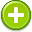basis 中文解釋 wordnet sense Collocation Usage
Noun
/ˈbāsis/,Font size:bases, plural;
1. The underlying support or foundation for an idea, argument, or process
• - trust is the only basis for a good working relationship
2. The system or principles according to which an activity or process is carried on
• - she needed coaching on a regular basis
• - flea markets operate on a cash-only basis
3. The justification for or reasoning behind something
• - on the basis of these statistics, important decisions are made

1. footing: a relation that provides the foundation for something; "they were on a friendly footing"; "he worked on an interim basis"
2. the fundamental assumptions from which something is begun or developed or calculated or explained; "the whole argument rested on a basis of conjecture"
3. the most important or necessary part of something; "the basis of this drink is orange juice"
4. In mineralogy and crystallography, crystal structure is a unique arrangement of atoms or molecules in a crystalline liquid or solid. A crystal structure is composed of a pattern, a set of atoms arranged in a particular way, and a lattice exhibiting long-range order and symmetry. ...
5. In linear algebra, a basis is a set of vectors that, in a linear combination, can represent every vector in a given vector space or free module, and such that no element of the set can be represented as a linear combination of the others. ...
6. In option trading, basis is used to evaluate the value differential between a call option and a put option. Also referred to as the reversal/conversion rate, it is calculated by determining the costs and benefits of being long or short the underlying security.
7. In mathematics, a base (or basis) B for a topological space X with topology T is a collection of open sets in T such that every open set in T can be written as a union of elements of B. We say that the base generates the topology T. ...
8. In universal algebra a basis is a structure inside of some (universal) algebras, which are called free algebras. It generates all algebra elements from its own elements by the algebra operations in an independent manner. ...
9. A starting point, base or foundation for an argument or hypothesis; An underlying condition or circumstance; In a vector space, a linearly independent set of vectors spanning the whole vector space; Amount paid for an investment, including commissions and other expenses; A collection of ...
10. An amount usually representing the actual cost of an investment to the buyer. The basis amount of an investment is important in calculating capital gains and losses, depreciation, and other income tax calculations.
11. The original cost and any additional outlays represent the cost basis in equity investments or property. The Internal Revenue Service computes the taxable gain, profit, or appreciation on the difference between the basis and the actual amount of sale. ...
12. The difference between the cash price and the futures price.
13. The starting point for computing gain or loss on a sale or exchange of property or for depreciation. (See "Adjusted basis.") For property that is purchased, basis is its cost. The basis of inherited property is its value at the date of death (or alternative valuation date). ...
14. Cost plus improvements less depreciation. Used to determine profit for tax purposes upon sale.
15. The starting point for determining gain or loss in any transaction. In general, basis is the cost of the taxpayer's property.
16. In the context of cubic splines, a basis is a set of cubic polynomials each of which is non-zero on a finite domain and such that any cubic spline can be calculated as a linear combination of cubics in the basis. Any cubic polynomial in the basis is referred to as a basis function.
17. The second segment of a thoracic limb. See pereopod.
18. The financial interest that the Internal Revenue Service attributes to an owner of an investment property for the purpose of determining annual depreciation and gain or loss on the sale of the asset. ...
19. an amount of money added to the NYMEX natural gas price to account for the pipeline and delivery costs of getting gas from the well-head to the local distribution center.
20. The value subtracted from the net sales price to calculate gain or loss for capital gains tax purposes.
21. The price an investor pays for a security plus any out-of-pocket expenses. It is used to determine capital gains or losses for tax purposes when the stock is sold.
22. A tax term, which refers to the original or acquisition value of a property, used to determine the amount of tax that will be assessed. The basis is deducted from the sales price of the property when it is sold to determine the profit or loss.
23. The benefactor s purchase price for an asset, possibly adjusted to reflect subsequent costs or depreciation. If Mrs. Quinn bought stock for \$100 per share and sold it for \$175, her cost basis in the stock is \$100 per share.
24. The original cost of the property plus capital improvements less accrued deprecation.
25. What you paid for an asset. The value that is used to determine gain or loss for income tax purposes.